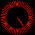## 2016年6月1日 星期三

### 給新手的C++教學 (上冊) - B. 遇到困難了？ (There are troubles?)

Q：

A：

(真的，不動手試試看就永遠學不會)

Q：

A：

Q：

A：

Q：

A：

#### 68 則留言:

1.啊抱歉排版跑掉了等我一下

2.和 一樣嗎是問那個stdio.h 和 cstdio

3.4.stdio.h 是給C程式語言用的
cstdio 是給C++程式語言用的
不知有沒有回答到您的問題？

5.有~!謝謝(可以刪了)

6.嗨，我現在懂了，我自以為
if(){
if(){}
}
else{}

if()
if(){}

else{}
一樣，結果下面變成
if(){
if(){}
else{}
}
所以就跑不出來

謝謝你

1.哈哈，我也犯過這種錯XD
所以會盡量避免這種寫法
寧願多加幾個大括號也不要最後debug一整天～OwO

7.你好，我是剛學沒幾天C++語言的初學者，你的教學淺顯易懂，非常感謝你寫這種教學文章 我有問題想要請教一下!!
#include
int main()
{
printf("我是天才?\n");
char n;
scanf("%c,&n");
if(n==是)
{
printf("真是識相!\n");
}
char b,c;
scanf("%c%c,&b,&c");
if(b,c==不是);
{
ptintf("你眼光有問題!\n");
}
return 0;
}
這個有錯誤 但是我不知道怎麼修正..

1.哈囉～其實您的程式碼有滿多錯誤的哦XD
舉幾個例子：
「scanf("%c,&n");」→「scanf("%c",&n);」（「"」位置放錯）
「if(n==是)」→「if(n=='是')」（請記得用「'」框住表示一個字元）
「ptintf("你眼光有問題!\n");」→「printf("你眼光有問題!\n");」（「printf」拼錯）
「if(b,c==不是);」→「if(b=='不'&&c=='是')」（修正邏輯、請記得用「'」框住表示一個字元、if後面直接分號=沒有作用(?)）
另外，有個重點是本教學忘記提及的，感謝您的提醒
「一個中文字」其實是「兩個字元」組成的哦～
因此就算只有「一個中文字」，也應該當作「字串」來看待
字串處理（中英文都是）其實還有有滿多需要注意的地方，是本教學有所遺漏的，小莫之後會將其加入，再次感謝您的發問！

2.我已經將你所舉的例子 訂正完了 不過還是有錯誤.

#include
int main()
{
printf("我是天才? YES or NO\n");
char n;
scanf("%c",&n);
if(n=='是')
{
ptintf("真是識相!\n");
}
char b,c;
scanf("%c%c,&b,&c");
if(b='不'&&c=='是')
{
ptintf("你眼光有問題!\n");
}
return 0;
}

3.還有 我有點看不太懂迴圈 1+2+3...+100 的那個例子

4.沒問題了-.-

5.執行的時候會停止運作.

6.我提到的只是一部分錯誤哦～
而且請注意到，一個中文字是兩個字元組成的，因此不能直接用「'」框起來（如之前的回答有誤導請見諒）
這裡提供我另外寫的程式碼，功能應該和您想實現的類似
可以修改看看並趁機將沒學好的觀念補回來哦～
另外，您有提到「執行的時候會停止運作」，請問是怎麼一回事呢？

#include<cstdio>
int main()
{
printf("我是天才? YES or NO\n");
{
printf("真是識相!\n");
}
{
printf("你眼光有問題!\n");
}
return 0;
}

7.8.請參考「陣列」這個章節哦～
網址：http://codingsimplifylife.blogspot.tw/2016/05/c-6-array.html

9.您好，已經針對您的問題做研究並撰寫教學囉～
給新手的C++教學 (上冊) - 13 - 7. 中文字元字串的處理
http://codingsimplifylife.blogspot.tw/2016/09/c-13-7.html

8.執行的時候 輸入後按ENTER就檔案停止運作

1.哈囉～請問是哪一份程式碼執行的時候按ENTER會檔案停止運作呢？
是教學內文的嗎？

9.不好意思想問一下

Finish the following requirements:
(1) Every time when a grade is inputted, print the grade and whether the grade is passed or not.
(2) Output the average (with one digits to the left of the decimal point) of all students.
(3) After finishing entering all grades, output the maximal grade among all.

我的問題是要怎麼寫可以隨意輸入數次的成績直到不想輸入了呢？然後就可以輸出平均及最大數
不太會寫QQ

謝謝~~

1.不知道可不可以給個提示或一開始應如何寫呢
謝謝~~

2.以下程式碼可做到：輸入任意個數字直到輸入不是數字(例如輸入「abc」)為止
#include<cstdio>
int main()
{
int n;
while(scanf("%d",&n)==1)
{
printf("n = %d\n",n);
}
printf("輸入完畢");
return 0;
}
若您想了解更多，scanf其實是一種函式，它的回傳值就是成功輸入的變數數量，可參考這篇：「scnaf 回傳值是 "引數成功 match 之數目"」

3.不好意思那如果用C++語法寫呢？
要怎麼寫判斷輸入的是否為數字呢？
(因為不太會用scanf及printf)QQ

4.在想是否可以用迴圈的寫法呢

5.抱歉小莫其實不太熟cin/cout XD
問過別人，可以這樣寫：
#include<iostream>
int main()
{
int n;
while(std::cin>>n)
{
std::cout<<"n = "<<n<<std::endl;
}
std::cout<<"輸入完畢";
return 0;
}
至於原理細節，會牽涉到覆載、進階轉型和許多小莫自己也不熟的概念。哈哈，有趣XD

10.請問一下
如果我想用define，這樣之後只要改一次數字的話
下面還要再宣告那個變數嗎

#define target 52
就是如果我打了上面
下面還需不需要打
int target
呢？

1.不用，可以直接用~
電腦會自動將程式碼裡面的每個「target」替換成「52」哦~

2.那如果再宣告一次可以嗎

3.不行哦，這樣等於是「int 52;」，並不符合語法(變數名稱不能用數字做開頭)，會編譯錯誤～

11.請問我想算
級數和 i=-1到i=1，(2i^3+6i=5)
code這樣寫
為什麼不對呢QQ

#include <iostream>
using namespace std;
int main(int argc, char **argv)
{
int k = 1, i, z;
for (int j = 1; j <= 3; j++)
{
k *= i;
}

for (i = -1; i <= 1; i++)
{
z = (2 * k + 6 * i + 5);
}

cout << z;
}

1.另外想問個問題
為什麼int k跟z都要在一開始就宣告呢？
如果我在for loop裡面才宣告k跟z
為什麼不行執行呢

2.啊啊抱歉我想更新一下問題
我發現我的一個錯誤了
但是似乎還有其他錯誤找不出來QQ

請問我想算
級數和 i=-2到i=10，〔2(i)^7+3i+7〕
code這樣寫
為什麼不對呢QQ

#include <iostream>
using namespace std;
int main(int argc, char **argv)
{
int k = 1, i, z, sum = 0;
for (int j = 1; j <= 7; j++)
{
k *= i;
}

for (i = -2; i <= 10; i++)
{
z = 2 * k + 3 * i + 7;
sum += z;
}

cout << sum;
}

另外還有個問題
為什麼int k跟z都要在一開始就宣告呢？
如果我在for loop裡面才宣告k跟z
為什麼不能執行呢

3.我有更新問題好像不見了QQ

4.您好，抱歉晚回
小莫大概知道您的問題出在哪了
那個k代表的是一個「變數(在這裡是指數字)」而不是「算式」哦
因此改變i並不會影響k的數值
關於您的第二個問題：
>為什麼int k跟z都要在一開始就宣告呢？
>如果我在for loop裡面才宣告k跟z
>為什麼不行執行呢
可以參考變數的作用範圍這篇哦～
最後，小莫已將您的程式碼修正，希望執行結果是您想要的！
#include <iostream>
using namespace std;
int main(int argc, char **argv)
{
int sum = 0;
for (int i = -2; i <= 10; i++)
{
int k = 1;
for (int j = 1; j <= 7; j++)
{
k *= i;
}
int z = (2 * k + 3 * i + 7);
sum += z;
}
cout << sum;
return 0;
}

5.那請問為什麼變數全部在外面宣告會不行呢
像這樣執行結果就不對QQ

#include <iostream>
using namespace std;
int main(int argc, char **argv)
{
int k = 1, i, z, sum = 0;

for (i = -2; i <= 10; i++)
{
for (int j = 1; j <= 7; j++)
{
k *= i;
}

z = (2 * k + 3 * i + 7);
sum += z;
}

cout << sum;
}

6.啊啊我知道是因為k的位置齁

12.想請問一下
這樣是不是會無法執行呢

#include <iostream>
using namespace std;

int A(int a)
{
return a;
}
int A(int a, int b = 2, int c = 3)
{
return a * b * c;
}

int main()
{
cout << A(4) << endl;
return 0;
}

1.是的，這樣電腦會分不清楚您的A(4)是要呼叫哪個函式哦～XD

2.那假設b跟c有一個沒有給預設值是不是就可以呢

3.恩恩，不過指定預設值的參數只能是最後連續幾個哦～

13.想請問以下這幾個的用法
查了一些資料但都不太清楚
快被搞昏頭了QQ
不知道小莫可不可以解釋一下或舉個例子><

1、cin
2、cin.get()
3、cin.getline()
4、getline()
5、gets()
6、getchar()

1.2.您好，可以參考這篇這篇哦～
看不懂歡迎再發問～ ^_^

3.不好意思想問一下
若我想輸入兩串資料
我用以下幾種寫法
但都會有些問題
不知道是為什麼呢？
要怎麼樣才能達到我想做的事呢？

(1)cin.get：
char a;
char b;
cin.get(a, 20);
cin.get(b, 20);
cout << endl;
cout << a << endl;
cout << b << endl;
問題：輸入完第一筆資料後，程式就自動停止輸出第一筆資料，無法輸入第二筆資料

(2)getline：
string a, b;
getline(cin, a);
getline(cin, a);
cout << a << endl;
cout << b << endl;
問題：輸入完兩筆資料，只會輸出第二筆資料

4.想再問個問題
我要開一個字串陣列
輸入資料到陣列並存起來
是只能用getline嗎？？
試了cin.get、cin.getline、gets好像都不適用字串陣列
不知道還有沒有其他方法呢？？
被這幾個輸入的語法搞到快瘋了����
感覺都很像����

5.能否麻煩您解答一下我的兩個問題呢><
謝謝~~

6.常常搞不懂哪個是用char，哪個是用string，哪個又可以用array QQ

7.8.QQQQQQ

14.int a1 = 1;
int *b1 = &a1;
cout << b1 << endl;

char a2 = 'A';
char *b2 = &a2;
cout << b2 << endl;

string a3 = "ABC";
string *b3 = &a3;
cout << b3 << endl;

不好意思想請問一下
我用int、char、string三種讀位址
但是為甚麼char型態跑出來的位址表示方法很奇怪呢(有些怪怪的符號)？？

1.真是有趣！
小莫研究了一下，發現如果用printf就沒事了呢XD
這應該是cout本身重載的問題哦～

#include<cstdio>
#include<iostream>
using namespace std;
int main()
{
int a1 = 1;
int *b1 = &a1;
cout << b1 << endl;
printf("0x%llx\n",b1);

char a2 = '\'';
char *b2 = &a2;
cout << b2 << endl;
printf("0x%llx\n",b2);

string a3 = "ABC";
string *b3 = &a3;
cout << b3 << endl;
printf("0x%llx\n",b3);

return 0;
}

2.是因為C裡面沒有string，char*會被當作 C style 的字串解讀，所以在印出 char* 的時候，C++ 會把 char* 所指向的位址上的資料以字串印出來嗎？？

3.因為用char arr[ ]這種型態輸出位址也會有這個問題
輸出的都會是資料字串而非位址

這是問了別人得到的解釋
不太知道有沒有錯呢

4.真有道理...是的cout會把型別為char*的東西當作字串來看待～所以才會出現如此令人匪夷所思的結果
謝謝你哦XD

15.我要轉換資料型態
除了static_cast (a)這種寫法外
如果要用簡單的寫法是
這種 (double) a
還是這種 double (a)
呢？？

1.是static_cast <double> (a)
打錯QQ

2.前者「(double)a」
本教學的這個章節有介紹到哦～ ^_^

16.17.不好意思
上面有個問cin的問題
可不可以回覆一下呢QQ><

1.不好意思，小莫對cin/cout這部分沒那麼熟，真抱歉>_<

18.#include
using namespace std;
int main(){
cout<<"hello world\n";//以iostream實作cin, cout測試例
string talk;
cout<<"input any string, plz."<>talk;
cout<>與<>，>>像人的手推拖板車，要裝卸給電腦。
cout或fout<<，<<像對話框的小尾巴，讓電腦說出來。

希望cstdio的I/O可以換用iostream, fstream的寫法比較物件導向。
不過cstdio如果有使用上較方便的I/O功能，也請多多說明、推廣。
感謝。
如果下冊要推廣STL，推薦參考以下這本：
https://github.com/yuanhui-yang/The-CPlusPlus-Standard-Library-A-Tutorial-and-Reference/blob/master/The%20C%2B%2B%20Standard%20Library%20-%20A%20Tutorial%20and%20Reference%20-%202nd%20Edition.pdf

1.#include < iostream > //漏打了*
...
cout<<"input any string, plz."<>talk;
} //被吃字的樣子*

2.cout << "input any string, plz." << endl;//吃字修正*
//吃字修正*
cin >> talk;//吃字修正*

3.被吃字的解決方法，請看這篇哦～
哪個比較方便，應該是看人啦XD (當時覺得scanf/printf的方式對新手應該較好理解)
您的比喻真生動，要不要考慮幫忙編輯教學文呢？因為小莫可能沒有空繼續寫下冊了>_<

19.#include
#include
int main()
{
for(int t=10;t>0;t--)
{
printf(" O\n");
printf("\n");
printf("I I\n");
sleep(1);
system("cls");
printf(" ");
}
return 0;
} 請問一下怎讓小人往又跑呢?我只有頭會動一下

1.您的code爛掉囉XD，請參考這篇來修正～
看不太懂您的程式碼耶......請問可以解釋一下嗎？><

20.我不是太明白[]的宣告和索引值的意思

1.宣告時[]裡面的數字代表你想開的陣列要多大（這個陣列裝了幾個元素）
宣告完之後[]裡面的數字就是索引值的意思，代表你想修改或讀取陣列中的哪一個元素
欸～～～這樣有清楚了嗎？有不懂的地方歡迎再發問哦！^_^